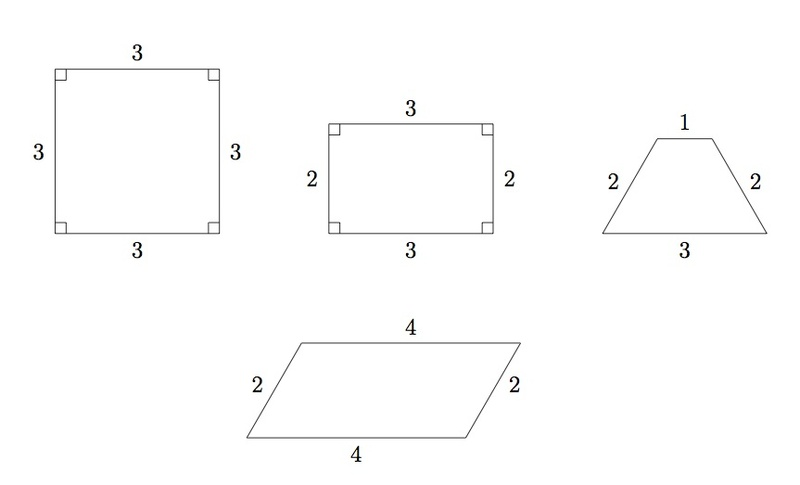# Lines of symmetry for quadrilaterals

Alignments to Content Standards: 4.G.A.3

Below are pictures of four quadrilaterals: a square, a rectangle, a trapezoid and a parallelogram.For each quadrilateral, find and draw all lines of symmetry.

## IM Commentary

This task provides students a chance to experiment with reflections of the plane and their impact on specific types of quadrilaterals. It is both interesting and important that these types of quadrilaterals can be distinguished by their lines of symmetry. The only pictures missing here, from this point of view, are those of a rhombus and a general quadrilateral which does not fit into any of the special categories considered here.

This task is best suited for instruction although it could be adapted for assessment. If students have not yet learned the terminology for trapezoids and parallelograms, the teacher can begin by explaining the meaning of those terms. 4.G.2 states that students should classify figures based on the presence or absence of parallel and perpendicular lines, so this task would work well in a unit that is addressing all the standards in cluster 4.G.A.

The students should try to visualize the lines of symmetry first, and then they can make or be provided with cutouts of the four quadrilaterals or trace them on tracing paper. It is useful for students to experiment and see what goes wrong, for example, when reflecting a rectangle (which is not a square) about a diagonal. This activity helps develop visualization skills as well as experience with different shapes and how they behave when reflected.

Students should return to this task both in middle school and in high school to analyze it from a more sophisticated perspective as they develop the tools to do so. In eighth grade, the quadrilaterals can be given coordinates and students can examine properties of reflections in the coordinate system. In high school, students can use the abstract definitions of reflections and of the different quadrilaterals to prove that these quadrilaterals are, in fact, characterized by the number of the lines of symmetry that they have.

## Solution

The lines of symmetry for each of the four quadrilaterals are shown below:When a geometric figure is folded about a line of symmetry, the two halves match up so if the students have copies of the quadrilaterals they can test lines of symmetry by folding. For the square, it can be folded in half over either diagonal, the horizontal segment which cuts the square in half, or the vertical segment which cuts the square in half. So the square has four lines of symmetry. The rectangle has only two, as it can be folded in half horizontally or vertically: students should be encouraged to try to fold the rectangle in half diagonally to see why this does not work. The trapezoid has only a vertical line of symmetry. The parallelogram has no lines of symmetry and, as with the rectangle, students should experiment with folding a copy to see what happens with the lines through the diagonals as well as horizontal and vertical lines.

The lines of symmetry indicated are the only ones for the figures. One way to show this is to note that for a quadrilateral, a line of symmetry must either match two vertices on one side of the line with two vertices on the other or it must pass through two of the vertices and then the other two vertices pair up when folded over the line. This limits the number of possible lines of symmetry and then experimentation will show that the only possible ones are those shown in the pictures.